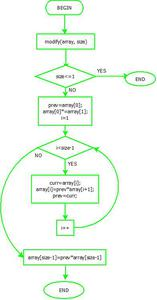# Replace every array element by multiplication of previous and next

• Difficulty Level : Easy
• Last Updated : 13 Sep, 2022

Given an array of integers, update every element with the multiplication of previous and next elements with the following exceptions.

a) The First element is replaced the by multiplication the of first and second.
b) The last element is replaced by multiplication of the last and second last.

Example:

```Input  : arr[] = {2, 3, 4, 5, 6}
Output : arr[] = {6, 8, 15, 24, 30}```
```// We get the above output using following
// arr[] = {2*3, 2*4, 3*5, 4*6, 5*6} ```
`Source: Top 25 Interview Questions`

Simple Solution is to create an auxiliary array ay, copy the contents the of given array to the auxiliary array. Finally, traverse the auxiliary array and update the given array using copied value. The timeTime complexity of this solution is O(n), but it requires O(n) extra space.
An efficient solution can solve the problem in O(n) time and O(1) space. The idea is to keep track of previous elements in the loop.

Flowchart:Flowchart- modify

Below is the implementation of this idea.

## C++

 `// C++ program to update every array element with``// multiplication of previous and next numbers in array``#include``using` `namespace` `std;` `void` `modify(``int` `arr[], ``int` `n)``{``    ``// Nothing to do when array size is 1``    ``if` `(n <= 1)``      ``return``;` `    ``// store current value of arr and update it``    ``int` `prev = arr;``    ``arr = arr * arr;` `    ``// Update rest of the array elements``    ``for` `(``int` `i=1; i

## Java

 `// Java program to update every array element with``// multiplication of previous and next numbers in array``import` `java.io.*;``import` `java.util.*;``import` `java.lang.Math;` `class` `Multiply``{``   ``static` `void` `modify(``int` `arr[], ``int` `n)``    ``{``        ``// Nothing to do when array size is 1``        ``if` `(n <= ``1``)``            ``return``;` `        ``// store current value of arr and update it``        ``int` `prev = arr[``0``];``        ``arr[``0``] = arr[``0``] * arr[``1``];` `        ``// Update rest of the array elements``        ``for` `(``int` `i=``1``; i

## Python3

 `# Python program to update every array element with``# multiplication of previous and next numbers in array` `def` `modify(arr, n):` ` ``# Nothing to do when array size is 1``    ``if` `n <``=` `1``:``        ``return` `    ``# store current value of arr and update it``    ``prev ``=` `arr[``0``]``    ``arr[``0``] ``=` `arr[``0``] ``*` `arr[``1``]` `    ``# Update rest of the array elements``    ``for` `i ``in` `range``(``1``, n``-``1``):``    ` `        ``# Store current value of next interaction``        ``curr ``=` `arr[i];` `        ``# Update current value using previous value``        ``arr[i] ``=` `prev ``*` `arr[i``+``1``]` `           ``# Update previous value``        ``prev ``=` `curr``    `  `    ``# Update last array element``    ``arr[n``-``1``] ``=` `prev ``*` `arr[n``-``1``]`  `# Driver program``arr ``=` `[``2``, ``3``, ``4``, ``5``, ``6``]``n ``=` `len``(arr)``modify(arr, n)``for` `i ``in` `range` `(``0``, n):``    ``print``(arr[i],end``=``" "``)` `# This code is contributed by``# Smitha Dinesh Semwal`

## C#

 `// C# program to update every array``// element with multiplication of``// previous and next numbers in array``using` `System;` `class` `GFG``{``    ``static` `void` `modify(``int` `[]arr, ``int` `n)``    ``{``        ``// Nothing to do when array size is 1``        ``if` `(n <= 1)``            ``return``;` `        ``// store current value of arr and update it``        ``int` `prev = arr;``        ``arr = arr * arr;` `        ``// Update rest of the array elements``        ``for` `(``int` `i=1; i

## PHP

 ``

## Javascript

 ``

Output

`6 8 15 24 30 `

Time complexity: O(n) where n is size of given array

Auxiliary Space: O(1) because it is using constant space for variables